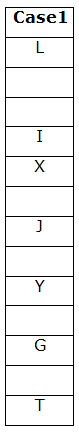# SBI Clerk Prelims Reasoning Ability Questions 2021 (Day-27)

Dear Aspirants, Our IBPS Guide team is providing new series of Reasoning Questions for SBI Clerk Prelims 2021 so the aspirants can practice it on a daily basis. These questions are framed by our skilled experts after understanding your needs thoroughly. Aspirants can practice these new series questions daily to familiarize with the exact exam pattern and make your preparation effective.

Start Quiz

Number series

Direction (1-4): Study the following information carefully and answer the below questions

528      439       671        264       863

1) If the numbers are written in descending order within the number from left to right, which of the following number will become the highest number?

A.863

B.264

C.671

D.439

E.528

2) If the second digit of each number is dropped, then the first and last digits of each number are interchanged, which of the following will be the lowest number?

A.863

B.528

C.671

D.439

E.264

3) If the position of the second and last digits are interchanged then how many numbers will be divisible by two?

A.1

B.2

C.3

D.4

E.none

4) What is the sum of the second digit ofthe highest number and the third digit of the lowest number?

A.10

B.14

C.12

D.8

E.6

Order and ranking

5) In a class, Raju is ranked 18th from the bottom and Mahesh is ranked 15th from the top. 8 persons have failed in at least one subject in the examination. If Raju is ranked immediately after Mahesh then how many students are in the class?

A.41

B.33

C.35

D.40

E.None of the above

Unknown box based puzzle

Direction (6-9): Study the following information carefully and answer the below questions.

A certain number of boxes are kept one above in the stack. Three boxes are kept between X and Y. Box I is exactly between Box J and Box L. Box I is kept immediately above Box X. The number of boxes above L is the same as below box T. Box Y is kept at an odd-number position when counts from both the top and bottom. Box G is placed two boxes below box Y. Box X has kept two boxes above Box J. Three boxes are there between T and Y. Only two boxes below G.

6) How many boxes are in the stack?

A.14

B.13

C.12

D.11

E.None of the above

7) What is the position of J from the bottom of the stack?

A.Seventh

B.Fifth

C.Sixth

D.Eighth

E.None of the above

8) How many boxes between L and Y?

A.Eight

B.Five

C.Seven

D.Six

E.None of the above

9) Which of the following statement is/are true?

I). Three boxes are between L and X

II). Box T is kept at the lowermost box

III). Box I is kept immediately above box X

A.Only I and III

B.Only III

C.Only I and II

D.Only II

E.None of the statement is false

Misc

10) Find the odd one out?

A.AG

B.JP

C.QV

D.SY

E.HN

Given series

528 439 671 264 863

After arranged in descending order from left to right

852  943 761 642 863

Given series

528 439 671 264 863

After the second digit dropped

58 49 61 24 83

After the First and last digits interchanged

85 94 16 42 38

Given series

528 439 671 264 863

Second and Last digits interchanged

582 493 617 246 836

Given series

528 439 671 264 863

6 + 4 = 10

18 + 15 + 8 = 41

Directions (6-9) :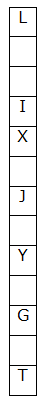Three boxes are kept between X and Y.

Box I is kept immediately above Box X.

From the above condition, there are two possibilities.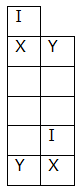Box G is placed two boxes below box Y.

Box X has kept two boxes above Box J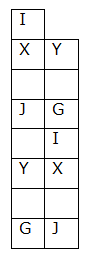Box I is exactly between Box J and Box L.

From the above condition, case2 gets eliminated.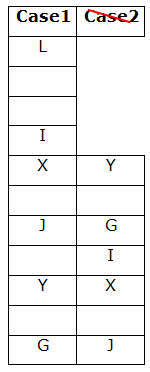The number of boxes above L is the same as below box T..Three boxes are there between T and Y. Only two boxes below G.

Box Y is kept at an odd-number position when counts from both the top and the bottom.

From the above condition, case1 shows the final arrangement.